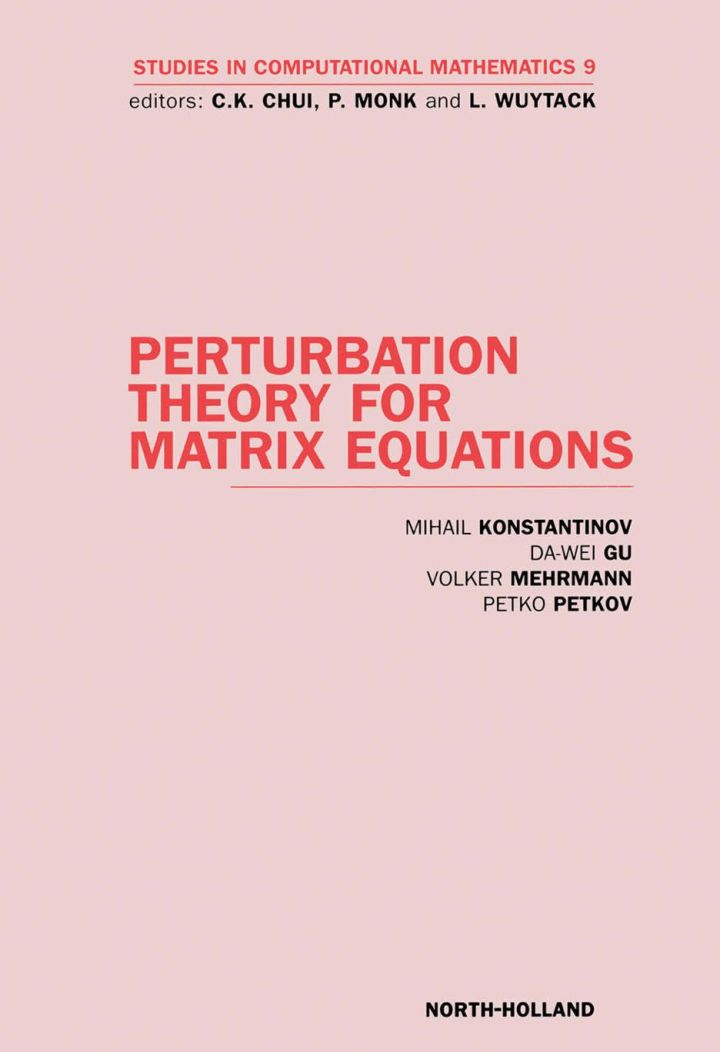# Perturbation Theory for Matrix Equations

## Perturbation Theory for Matrix Equations

By Konstantinov, M.; Wei Gu, D.; Mehrmann, V.; Petkov, P.

### Rent or Buy eTextbook

Expires on Apr 16th, 2022
\$225

Publisher List Price: \$229.99
Savings: \$4.99

The book is devoted to the perturbation analysis of matrix equations. The importance of perturbation analysis is that it gives a way to estimate the influence of measurement and/or parametric errors in mathematical models together with the rounding errors done in the computational process. The perturbation bounds may further be incorporated in accuracy estimates for the solution computed in finite arithmetic. This is necessary for the development of reliable computational methods, algorithms and software from the viewpoint of modern numerical analysis.

In this book a general perturbation theory for matrix algebraic equations is presented. Local and non-local perturbation bounds are derived for general types of matrix equations as well as for the most important equations arising in linear algebra and control theory. A large number of examples, tables and figures is included in order to illustrate the perturbation techniques and bounds.

Key features:

• The first book in this field
• Can be used by a variety of specialists
• Material is self-contained
• Results can be used in the development of reliable computational algorithms
• A large number of examples and graphical illustrations are given
• Written by prominent specialists in the field

Subject: Allied Health & Medical -> Medical -> GeneralPublisher: Elsevier S & T 05/2003
Imprint: JAI Press
Language: English
Length: 442 pages

ISBN 10: 0444513159
ISBN 13: 9780444513151
Print ISBN: 9780444513151

Live Chats